Hostname: page-component-5db6c4db9b-wnbrb Total loading time: 0 Render date: 2023-03-27T02:32:00.199Z Has data issue: true Feature Flags: { "useRatesEcommerce": false } hasContentIssue true

# Existence of bounded solutions of some nonlinear parabolic equations

Published online by Cambridge University Press:  14 November 2011

## Synopsis

This paper proves the existence of (at least) one solution of the following equation: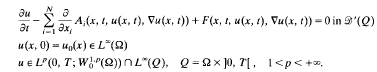Here,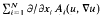is an elliptic operator of Leray-Lions type acting from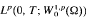into Lp′(0, T; W−1.p′ (Ω)), (1/p + 1/p′ = 1) and |F(u, ∇u)| ≧b(|u|)(l + |≧u|P). There are no smoothness assumptions on the bounded open set Ω; the operator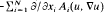and the nonlinearity F(u, ∇u) are denned in terms of Carathéodory functions. These points are the most characteristic features of this paper.

Assuming the existence of upper and lower solutions allows us to obtain L(Q)-estimates. An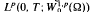estimate is then proved. The final step is to prove the strong convergence inof the approximations. This proof relies on the method introduced by L. Boccardo, F. Murat and J. P. Puel for elliptic and parabolic problems of this type.

Type
Research Article

## Access options

Get access to the full version of this content by using one of the access options below. (Log in options will check for institutional or personal access. Content may require purchase if you do not have access.)

## References

1Amann, H.. Periodic solutions of semi-linear parabolic equations. Nonlinear Analysis, a collection of papers in honor of E. H. Rothe, eds Cesari, L., Kannan, R. and Weinberger, H. F., pp. 129 (New York: Academic Press, 1978).Google Scholar
2Boccardo, L., Murat, F. and Puel, J. P.. Existence de solutions faibles pour des équations elliptiques quasilinéaires à croissance quadratique. Nonlinear partial differential equations and their applications, Collège de France Seminar, Vol. IV, eds. Brézis, H. and Lions, J. L., pp. 1973. Research Notes in Mathematics 84 (London: Pitman, 1983).Google Scholar
3Boccardo, L., Murat, F. and Puet, J. P.. Existence de solutions non bornées pour certaines équations quasilinéaires. Portugal. Math. 41 (1982) (volume dedicated to J. Sebastioa E Silva), 507534.Google Scholar
4Boccardo, L., Murat, F. and Puet, J. P.. Résultats d'existence pour certains problemes elliptiques quasilinéaires. Ann. Scuola Norm. Sup. Pisa Cl. Sci. (4) 11 (1984), 213235.Google Scholar
5Boccardo, L., Murat, F. and Puel, J.. Existence results for some quasilinear parabolic equations (to appear).Google Scholar
6Boccardo, L., Murat, F. and Puel, J.. Existence of bounded solutions for nonlinear elliptic unilateral problems (to appear).Google Scholar
7Browder, F. E.. Existence theorems for nonlinear partial differential equations. Proceedings of Symposia in Pure Mathematics, Vol. XVI, eds. Chern, S. and Smale, S., pp. 160 (Providence: American Mathematical Society, 1970).Google Scholar
8Deuel, J. and Hess, P.. Nonlinear parabolic boundary value problems with upper and lower solutions. Israel J. Math. 29 (1978), 92104.CrossRefGoogle Scholar
9Frehse, J.. Existence results for parabolic nonlinear problems (to appear).Google Scholar
10Hess, P.. On a second order nonlinear elliptic boundary value problem. Nonlinear Analysis, a collection of papers in honor of E. H. Rothe, eds. Cesari, L., Kannan, R. and Weinberger, H. F., pp. 99107 (New York: Academic Press, 1978).Google Scholar
11Ladyzenskaya, O. A. and Uralceva, N. N.. Linear and Quasilinear Elliptic Equations (New York: Academic Press, 1968).Google Scholar
12Lions, J. L.. Quelques Méthodes de Résolution des Problèmes aux Limites non Linéaires (Paris: Dunod, 1969).Google Scholar
13Puel, J. P.. Existence, comportement à l'infini et stabilité dans certains problèmes quasilinéaires elliptiques et paraboliques d'ordre 2. Ann. Scuola Norm. Sup. Pisa 3 (1976), 89119.Google Scholar
14Puel, J. P.. A compactness theorem in quasilinear parabolic problems and application to an existence results. Proceedings of the meeting on parabolic problem, Rome April 1985. Research Notes in Mathematics (London: Pitman, to appear).Google Scholar
15Simon, J.. Ecoulement d'un fluide non homogène avec une densité initiale s'annulant. C.R. Acad. Sci. Paris 287 (1978), 10091012.Google Scholar
16Simon, J.. Compact sets in the space L p(0, T; B). Ann. Math. Pura Appl. (to appear).Google Scholar
17Stampacchia, G.. Equations elliptiques du second ordre à coefficients discontinus. Séminaire de Mathématiques Supérieures 16 (Montreal: Les Presses de l'Universite de Montreal, 1966).Google Scholar
18Temam, R.. Navier-Stokes Equations. (Amsterdam: North-Holland, 1977).Google Scholar